Anzeige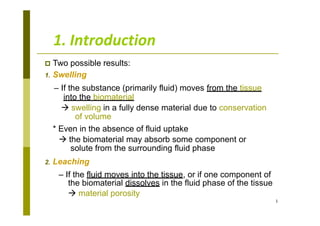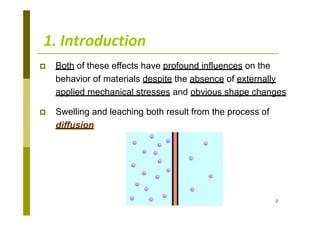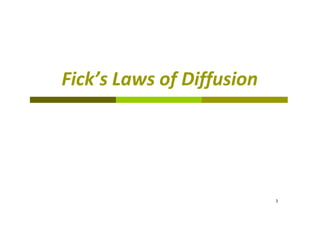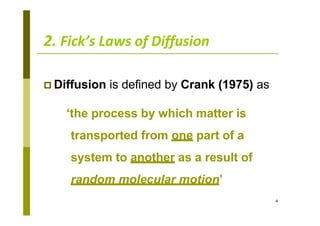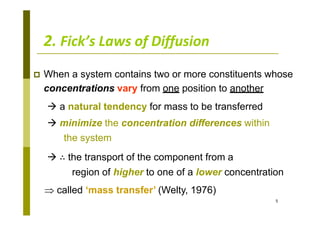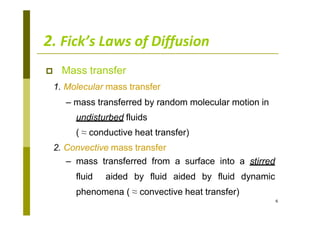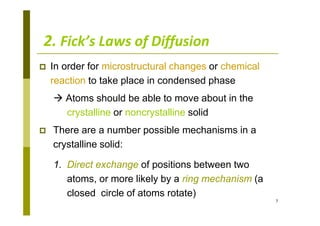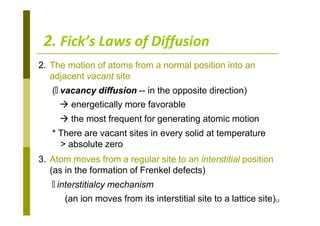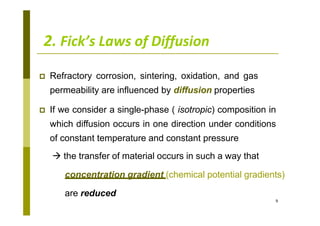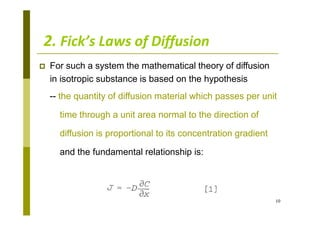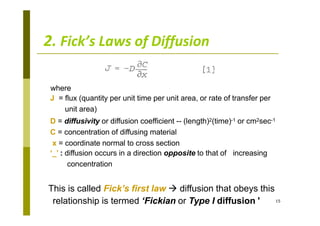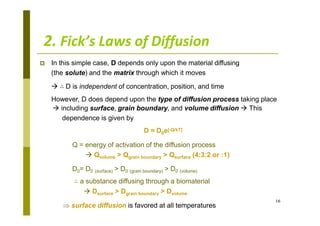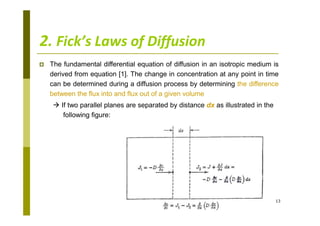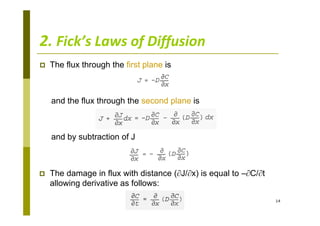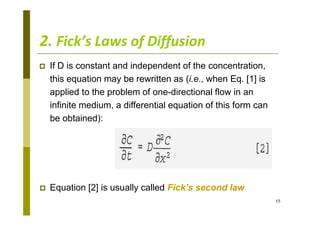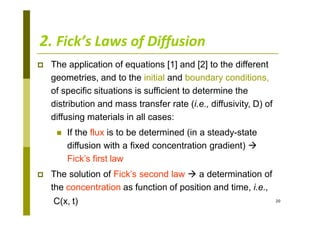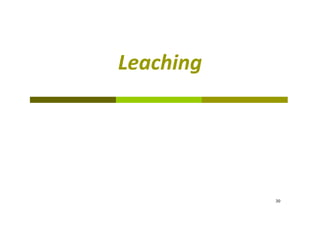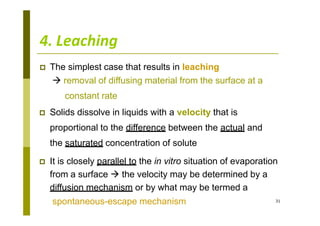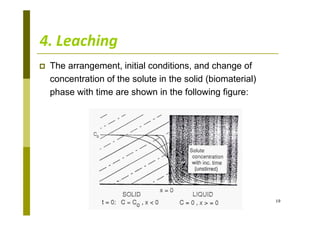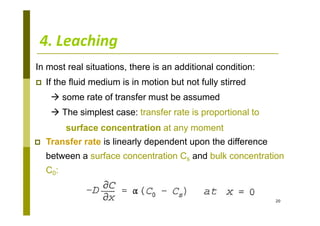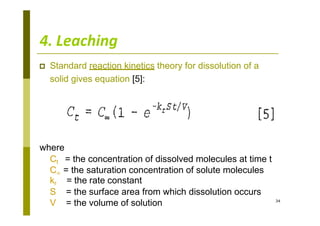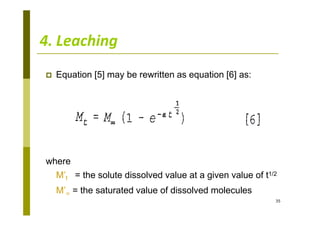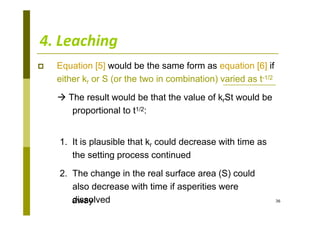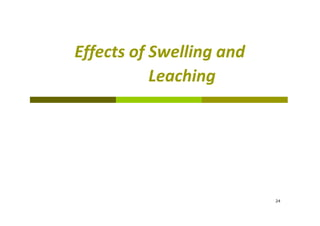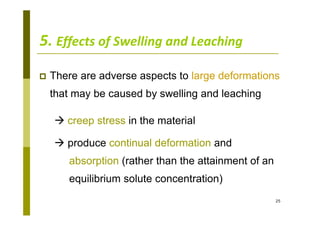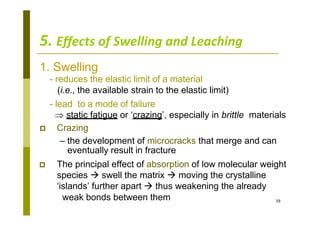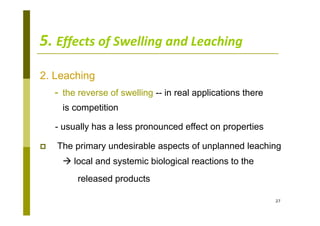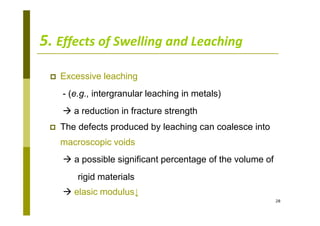1 von 28
Anzeige

### SWELLING AND LEACHING.pptx

1. 1. Introduction 1  Two possible results: 1. Swelling – If the substance (primarily fluid) moves from the tissue into the biomaterial  swelling in a fully dense material due to conservation of volume * Even in the absence of fluid uptake  the biomaterial may absorb some component or solute from the surrounding fluid phase 2. Leaching – If the fluid moves into the tissue, or if one component of the biomaterial dissolves in the fluid phase of the tissue  material porosity
2. 1. Introduction  Both of these effects have profound influences on the behavior of materials despite the absence of externally applied mechanical stresses and obvious shape changes  Swelling and leaching both result from the process of diffusion 2
3. Fick’s Laws of Diffusion 3
4. 2. Fick’s Laws of Diffusion 4  Diffusion is defined by Crank (1975) as ‘the process by which matter is transported from one part of a system to another as a result of random molecular motion’
5. 2. Fick’s Laws of Diffusion 5  When a system contains two or more constituents whose concentrations vary from one position to another  a natural tendency for mass to be transferred  minimize the concentration differences within the system  ∴ the transport of the component from a region of higher to one of a lower concentration  called ‘mass transfer’ (Welty, 1976)
6. 2. Fick’s Laws of Diffusion 6  Mass transfer 1. Molecular mass transfer – mass transferred by random molecular motion in undisturbed fluids ( ≈ conductive heat transfer) 2. Convective mass transfer – mass transferred from a surface into a stirred fluid aided by fluid aided by fluid dynamic phenomena ( ≈ convective heat transfer)
7. 2. Fick’s Laws of Diffusion 7  In order for microstructural changes or chemical reaction to take place in condensed phase  Atoms should be able to move about in the crystalline or noncrystalline solid  There are a number possible mechanisms in a crystalline solid: 1. Direct exchange of positions between two atoms, or more likely by a ring mechanism (a closed circle of atoms rotate)
8. 2. The motion of atoms from a normal position into an adjacent vacant site (🡘 vacancy diffusion -- in the opposite direction)  energetically more favorable  the most frequent for generating atomic motion * There are vacant sites in every solid at temperature > absolute zero 3. Atom moves from a regular site to an interstitial position (as in the formation of Frenkel defects) 🡘 interstitialcy mechanism (an ion moves from its interstitial site to a lattice site)12 2. Fick’s Laws of Diffusion
9. 2. Fick’s Laws of Diffusion 9  Refractory corrosion, sintering, oxidation, and gas permeability are influenced by diffusion properties  If we consider a single-phase ( isotropic) composition in which diffusion occurs in one direction under conditions of constant temperature and constant pressure  the transfer of material occurs in such a way that concentration gradient (chemical potential gradients) are reduced
10. 2. Fick’s Laws of Diffusion  For such a system the mathematical theory of diffusion in isotropic substance is based on the hypothesis -- the quantity of diffusion material which passes per unit time through a unit area normal to the direction of diffusion is proportional to its concentration gradient and the fundamental relationship is: 10
11. 15 2. Fick’s Laws of Diffusion where J = flux (quantity per unit time per unit area, or rate of transfer per unit area) D = diffusivity or diffusion coefficient -- (length)2(time)-1 or cm2sec-1 C = concentration of diffusing material x = coordinate normal to cross section ‘_’ : diffusion occurs in a direction opposite to that of increasing concentration This is called Fick’s first law  diffusion that obeys this relationship is termed ‘Fickian or Type I diffusion '
12. 16 2. Fick’s Laws of Diffusion  In this simple case, D depends only upon the material diffusing (the solute) and the matrix through which it moves  ∴ D is independent of concentration, position, and time However, D does depend upon the type of diffusion process taking place  including surface, grain boundary, and volume diffusion  This dependence is given by D = D0e[-Q/kT] Q = energy of activation of the diffusion process  Qvolume > Qgrain boundary > Qsurface (4:3:2 or :1) D0= D0 (surface) > D0 (grain boundary) > D0 (volume) ∴ a substance diffusing through a biomaterial  Dsurface > Dgrain boundary > Dvolume  surface diffusion is favored at all temperatures
13. 2. Fick’s Laws of Diffusion  The fundamental differential equation of diffusion in an isotropic medium is derived from equation . The change in concentration at any point in time can be determined during a diffusion process by determining the difference between the flux into and flux out of a given volume  If two parallel planes are separated by distance dx as illustrated in the following figure: 13
14. 2. Fick’s Laws of Diffusion  The flux through the first plane is and the flux through the second plane is and by subtraction of J  The damage in flux with distance (J/x) is equal to –C/t allowing derivative as follows: 14
15. 2. Fick’s Laws of Diffusion  If D is constant and independent of the concentration, this equation may be rewritten as (i.e., when Eq.  is applied to the problem of one-directional flow in an infinite medium, a differential equation of this form can be obtained):  Equation  is usually called Fick’s second law 15
16. 20 2. Fick’s Laws of Diffusion  The application of equations  and  to the different geometries, and to the initial and boundary conditions, of specific situations is sufficient to determine the distribution and mass transfer rate (i.e., diffusivity, D) of diffusing materials in all cases:  If the flux is to be determined (in a steady-state diffusion with a fixed concentration gradient)  Fick’s first law  The solution of Fick’s second law  a determination of the concentration as function of position and time, i.e., C(x, t)
17. 30 Leaching
18. 31 4. Leaching  The simplest case that results in leaching  removal of diffusing material from the surface at a constant rate  Solids dissolve in liquids with a velocity that is proportional to the difference between the actual and the saturated concentration of solute  It is closely parallel to the in vitro situation of evaporation from a surface  the velocity may be determined by a diffusion mechanism or by what may be termed a spontaneous-escape mechanism
19. 4. Leaching  The arrangement, initial conditions, and change of concentration of the solute in the solid (biomaterial) phase with time are shown in the following figure: 19
20. 4. Leaching In most real situations, there is an additional condition:  If the fluid medium is in motion but not fully stirred  some rate of transfer must be assumed  The simplest case: transfer rate is proportional to surface concentration at any moment  Transfer rate is linearly dependent upon the difference between a surface concentration Cs and bulk concentration C0: 20
21. 34 4. Leaching  Standard reaction kinetics theory for dissolution of a solid gives equation : where Ct = the concentration of dissolved molecules at time t C∞ = the saturation concentration of solute molecules kr = the rate constant S = the surface area from which dissolution occurs V = the volume of solution
22. 35 4. Leaching  Equation  may be rewritten as equation  as: where M’t = the solute dissolved value at a given value of t1/2 M’∞ = the saturated value of dissolved molecules
23. 36 4. Leaching  Equation  would be the same form as equation  if either kr or S (or the two in combination) varied as t-1/2  The result would be that the value of krSt would be proportional to t1/2: 1. It is plausible that kr could decrease with time as the setting process continued 2. The change in the real surface area (S) could also decrease with time if asperities were dissolved away
24. Effects of Swelling and Leaching 24
25. 5. Effects of Swelling and Leaching 25  There are adverse aspects to large deformations that may be caused by swelling and leaching  creep stress in the material  produce continual deformation and absorption (rather than the attainment of an equilibrium solute concentration)
26. 39 5. Effects of Swelling and Leaching 1. Swelling - reduces the elastic limit of a material (i.e., the available strain to the elastic limit) - lead to a mode of failure  static fatigue or ‘crazing’, especially in brittle materials  Crazing – the development of microcracks that merge and can eventually result in fracture  The principal effect of absorption of low molecular weight species  swell the matrix  moving the crystalline ‘islands’ further apart  thus weakening the already weak bonds between them
27. 5. Effects of Swelling and Leaching 27 2. Leaching - the reverse of swelling -- in real applications there is competition - usually has a less pronounced effect on properties  The primary undesirable aspects of unplanned leaching  local and systemic biological reactions to the released products
28. 5. Effects of Swelling and Leaching 28  Excessive leaching - (e.g., intergranular leaching in metals)  a reduction in fracture strength  The defects produced by leaching can coalesce into macroscopic voids  a possible significant percentage of the volume of rigid materials  elasic modulus↓
Anzeige